When calculating the combustion flow problem, in addition to calculating the flow, it is also necessary to consider the chemical reactions between the components. In the actual combustion process, there will be a large number of different chemical reactions, and the reaction rate between the different chemical reactions may differ by several orders of magnitude, thus causing greater trouble for the accurate numerical solution of combustion. This example uses commercial software to solve the combustion of methane premixed gas, which provides comparative data for the development of subsequent independent programs. The geometric model during calculation is shown in Figure 1, and the schematic diagram of the grid is shown in Figure 2: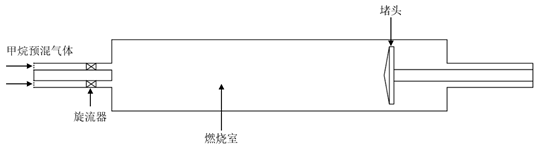Figure 1. Geometric Model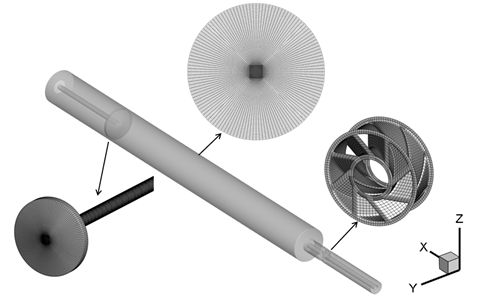Figure 2. Schematic Diagram of Grid

In this example, a large eddy simulation is used for calculation, and a simplified two-step reaction is used for the chemical reaction: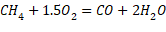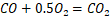The equivalent ratio of the premixed gas at the inlet is 0.6, the speed is 42 m/s, the pressure is 1 atm, the temperature is 200°C, and the pressure at the outlet is 1 atm. The wall surface other than the plug is set as an insulated wall surface, the temperature of the plug is set to 300k, and the heat transfer coefficient is 200W/(m2·K). The calculated time step is 5×10-5s. Before the LES unsteady calculation, the flow and temperature fields are calculated to a steady state using the RANS model.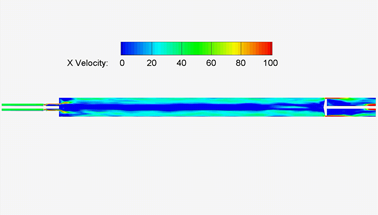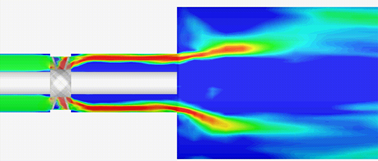Figure 3. Instantaneous flow velocity distribution in longitudinal section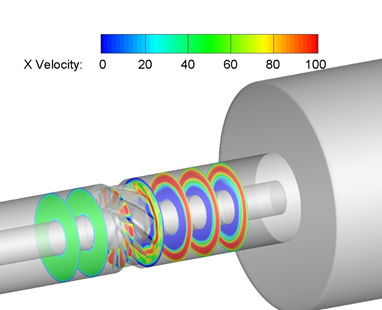Figure 4. Instantaneous flow velocity distribution in cross-section

Figures 3 and 4 are the instantaneous flow velocity distribution diagrams of the longitudinal section and the transverse section. It can be seen from the figure that after the airflow passes through the cyclone, the radial outer acceleration accelerates to form a high-speed region, while the inner airflow returns. In practical applications, this situation is unfavorable for the cyclone, so the position and related geometry of the cyclone need to be adjusted. After entering the combustion chamber, the presence of the recirculation zone increases the stability of the flame. Figure 5 shows the instantaneous temperature distribution diagram of the longitudinal section. From the figure, it can be seen that after passing through the cyclone, a high temperature zone is formed on the radially inner side, and a low temperature zone is formed on the outer side. This is because the airflow passes through the cyclone Afterwards, the centrifugal effect of the swirl flow makes the unburned gas with high density move from inside to outside under the action of centrifugal force, while the flammable gas with low density moves from outside to inside.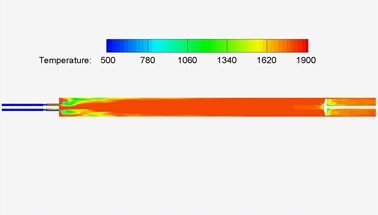Figure 5. Instantaneous Temperature distribution in cross-section